Midterm 3 Preparation and Sample Questions

# Midterm 3: Version A

For problems 1–4, perform the indicated operations and simplify.

1.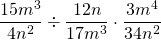2.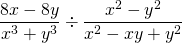3.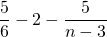4.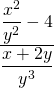Reduce the expressions in questions 5–7.

1.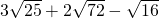2.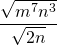3.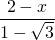Solve for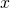.

1.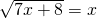For problems 9–12, find the solution set by any convenient method.

1.1.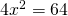2.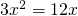2.1.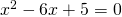2.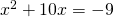3.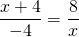4.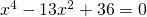5. The base of a right triangle is 10 m longer than its height. If the area of this triangle is 300 m2, what are its base and height measurements?
6. Find three consecutive odd integers such that the product of the first and the third is 38 more than the second.
7. Two airplanes take off from the same airfield, with the first plane leaving at 6 a.m. and the second at 7:30 a.m. The second airplane, travelling at 150 km/h faster than the first, catches up to the first plane by 10:30 a.m. What is the speed of each airplane?